Ask your WordPress questions! Pay money and get answers fast!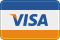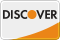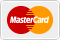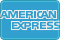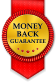# Combine 2 arrays to 1 WordPress

• SOLVED

How to combine 2 arrays to 1?

I have two arrays:

```Array (  => stdClass Object ( [name] => John [id] => 1 ) } Array (  => stdClass Object ( [meta_value] => 9 [id] => 1 ) }```

I need to get:

```Array (  => stdClass Object ( [meta_value] => 9 [name] => John [id] => 1 ) }```

2012-05-25

Try this simple way

``` <?php \$array_a = array(0 => array( 'name' => 'John', 'id' => 1 )); \$array_b = array(0 => array( 'meta_value' => 9, 'id' => 1 )); \$array_c = array(); foreach (\$array_a as \$key => \$subarray) { foreach(\$subarray as \$name => \$value) { \$array_c[\$key][\$name] = \$value; } } foreach (\$array_b as \$key => \$subarray) { foreach(\$subarray as \$name => \$value) { \$array_c[\$key][\$name] = \$value; } } print_r( \$array_c ); ```

Hi Luis,

Thank you! That almost works for me.

However I've got <strong>Array</strong> instead of <strong>stdClass Object</strong>:

Array (

 => Array (

[meta_value] => 9

[name] => John

[id] => 1

)

}

Array (

 => stdClass Object (

[meta_value] => 9

[name] => John

[id] => 1

)

}

How to get a correct result?

Change the array for an stdClass

``` <?php \$array_a = array(0 => array( 'name' => 'John', 'id' => 1 )); \$array_b = array(0 => array( 'meta_value' => 9, 'id' => 1 )); \$array_c = new stdClass(); foreach (\$array_a as \$key => \$subarray) { foreach(\$subarray as \$name => \$value) { \$array_c->{\$name} = \$value; } } foreach (\$array_b as \$key => \$subarray) { foreach(\$subarray as \$name => \$value) { \$array_c->{\$name} = \$value; } } print_r( \$array_c ); ```

Now I've got a

```stdClass Object ( [meta_value] => 9 [name] => Metal Professionals [id] => 1 ) ```

But I need a

```Array (  => stdClass Object ( [meta_value] => 9 [name] => Metal Professionals [id] => 1 )```

Ohh i see, just add the object to an array,

``` <?php \$array_a = array(0 => array( 'name' => 'John', 'id' => 1 )); \$array_b = array(0 => array( 'meta_value' => 9, 'id' => 1 )); \$array_c = array(); foreach (\$array_a as \$key => \$subarray) { \$array_c[\$key] = new stdClass(); foreach(\$subarray as \$name => \$value) { \$array_c[\$key]->{\$name} = \$value; } } foreach (\$array_b as \$key => \$subarray) { foreach(\$subarray as \$name => \$value) { \$array_c[\$key]->{\$name} = \$value; } } print_r( \$array_c ); ```

This way

``` <?php \$array_a = array(0 => array( 'name' => 'John', 'id' => 1 )); \$array_b = array(0 => array( 'meta_value' => 9, 'id' => 1 )); \$array_c = array(); foreach (\$array_a as \$key => \$subarray) { \$array_c[\$key] = new stdClass(); foreach(\$subarray as \$name => \$value) { \$array_c[\$key]->{\$name} = \$value; } } foreach (\$array_b as \$key => \$subarray) { if (!is_object(\$array_c[\$key])) { \$array_c[\$key] = new stdClass(); } foreach(\$subarray as \$name => \$value) { \$array_c[\$key]->{\$name} = \$value; } } print_r( \$array_c ); ```

<strong>BINGO! Thats that I need!</strong>

That works! Amazing!

Luis,

Thank you so much for your time. I'm really appreciate your help.

PS: Can I doubled the bet for another small question? Is there way to sort <strong>\$array_c</strong> by <strong>meta_value</strong> key value? I mean DESC order.

I've found a solution.

```function cmp(\$a, \$b) { if (\$a->meta_value == \$b->meta_value) : return 0; else : return \$a->meta_value < \$b->meta_value ? 1 : -1; endif; } usort(\$array_c, 'cmp');```

Luis,

Thanks again!

2012-05-25

use this
<?php \$result = array_unique(array_merge(\$array1, \$array2)); ?>

Hi Arnav,

Unfortunately, I've got an error:

<strong>Catchable fatal error: Object of class stdClass could not be converted to string</strong>

try this

<?php

\$array1 = array(0 => array(

'name' => 'John',

'id' => 1

));

\$array2 = array(0 => array(

'meta_value' => 9,

'id' => 1

));

\$array3 = new stdClass();

foreach (\$array1 as \$k => \$val) {

foreach(\$val as \$key => \$value) {

\$array3->{\$key} = \$value;

}

}

foreach (\$array2 as \$k => \$val) {

foreach(\$val as \$key => \$value) {

\$array3->{\$key} = \$value;

}

}

\$arr = \$array3 ;

print_r( \$arr );

?>

2012-05-25

<?php
\$array1 = array();
\$array2 = array();
\$result = array_merge(\$array1, \$array2);
?>

2012-05-25

You have: array_a and array_b, well:

``` array_new = array(); for(\$i = 0; \$i < count(\$array_a); \$i++) { array_new[] = array_unique(array_merge(\$array_a[\$i], \$array_b[\$i])); } ```

Hope this helps!

You have: array_a and array_b, well:

``` array_new = array(); for(\$i = 0; \$i < count(\$array_a); \$i++) { array_new[] = array_unique(array_merge(\$array_a[\$i], \$array_b[\$i])); } ```

Hope this helps!

2012-05-25

try array_merge i think this is the function to achieve your target array

http://www.w3schools.com/php/func_array_merge.asp

2012-05-25

Or you can simply add to arrays like:

`\$combine_array = \$array1 + \$array2; `

2012-05-25

`\$merged = array( (object) array_merge((array) \$array1, (array) \$array2) );`
I created the the arrays you wanted to merge and tested the script. Screenshot: [[LINK href="http://cl.ly/Gttn"]]http://cl.ly/Gttn[[/LINK]]

Kailey,

Thank you for a reply. That almost works for me. Is there way to get result by the same way in case I have a few arrays on each sides?

e.g.

```Array (  => stdClass Object ( [name] => John [id] => 1 ) } Array (  => stdClass Object ( [name] => Mark [id] => 2 ) } ```

```Array (  => stdClass Object ( [meta_value] => 9 [id] => 1 ) } Array (  => stdClass Object ( [meta_value] => 10 [id] => 2 ) }```

I'm assuming an key of 0 in my little script.

Depending on how many you're dealing with, you can run the script a few times and manually change the keys:
`\$merged = array( (object) array_merge((array) \$array1, (array) \$array2) );`

If you have a whole bunch, you can run it through a loop. I'm assuming 20 below:
``` for( \$i = 0; \$i <= 20; ++\$i ) { \$merged = array( (object) array_merge((array) \$array1[ \$i ], (array) \$array2[ \$i ]) ); } ```

Are you dealing with a lot of variables, each containing an array with an object? Or just one variable containing an array of arrays each containing an object?

``` \$merged = array(); for( \$i = 0; \$i <= 20; ++\$i ) { \$merged[] = array( (object) array_merge((array) \$array1[ \$i ], (array) \$array2[ \$i ]) ); } ```